• 不同维度的特征
2018-12-14 23:13:11

1 维度压缩

主成分分析（rincipal components Analysis）

在减少数据集的维度的同时，保持对方差贡献最大的特征

2 PCA方法

pca_3=PCA(n_components=3) 维度设置

fit 训练数据

data_pca_3=pca_3.fit_transform(data)

3 代码案例事例


import pandas
from sklearn import datasets
import matplotlib.pyplot as plt
from sklearn.decomposition import PCA
from mpl_toolkits.mplot3d import Axes3D

# d加载库里边的iris数据集

# 选择自变量和因变量

data=iris.data
target=iris.target

# 选择维度并进行训练

pca_3=PCA(n_components=3)

data_pca_3=pca_3.fit_transform(data)

"""
散点样式：

参数	样式
'.'	实心点
'o'	圆圈
','	一个像素点
'x'	叉号
'+'	十字
'*'	星号
'^' 'v' '<' '>'	三角形(上下左右)
'1' '2' '3' '4'	三叉号（上下左右）

颜色：

参数	颜色
'b'	蓝
'g'	绿
'r'	红
'c'	青
'm'	品红
'y'	黄
'k'	黑
'w'	白
"""

### 定义颜色和三点样式

colors={
0:'r',
1:'b',
2:'k'
}

markers={
0:'X',
1:'D',
2:'o'
}

# 弹出图形 %matplotlib qt

# 调用三维数据绘图方法
# # figure 3，指定figure的编号并指定figure的大小,

# 指定线的颜色, 宽度和类型

fig=plt.figure(1,figsize=(8,6))

ax=Axes3D(fig,elev=-150,azim=110)

# 对降维后的数据进行分组

data_pca_gb = pandas.DataFrame(
data_pca_3
).groupby(by=target)

# 用数据调用scatter方法进行绘图

for g in data_pca_gb.groups:
ax.scatter(
data_pca_gb.get_group(g), # x轴
data_pca_gb.get_group(g),
data_pca_gb.get_group(g),
c=colors[g],
marker=markers[g],
cmap=plt.cm.Paired
)

#二维数据
pca_2 = PCA(n_components=2)
data_pca_2 = pca_2.fit_transform(data)

data_pca_gb = pandas.DataFrame(data_pca_2).groupby(target)

import matplotlib.pyplot as plt;

for g in data_pca_gb.groups:
plt.scatter(
data_pca_gb.get_group(g),
data_pca_gb.get_group(g),
c=colors[g],
marker=markers[g]
)



更多相关内容
• 2维线性判别2D-LDA_输出不同维度特征的识别率曲线.
• 特征如：颜色特征、SIFT特征、HOG特征、HOF特征、GIST特征等 分类器如：KNN、SVM，Random Forest、CNN等 特征分为：人工设计的特征 和 深度特征，前者特点鲜明意义明确，后者面向具体问题（即理解为 提取的特征 是...

• 多维度是数学上的，即张量
• 多模态是数据类型上的，比如音频，视频，图像，传感器回传数值

# 模式分类中的特征融合方法

## 引入

• 传统模式识别思路：特征工程（特征提取、特征降维、特征融合等）、分类器设计、训练测试
• 特征如：颜色特征、SIFT特征、HOG特征、HOF特征、GIST特征等
• 分类器如：KNN、SVM，Random Forest、CNN等
• 特征分为：人工设计的特征 和 深度特征，前者特点鲜明意义明确，后者面向具体问题（即理解为 提取的特征 是针对CNN训练时侧重的具体问题和场景 ）
• 一般 特征存在：类内方差小 而 类间方差大（即不同样本的同种特征的差异小，而不同的特征的差异大），所以从这里就可以知道 多模态的特征 融合 不能是 简单的相加相减或者拼接就完事了，会导致可能融合的特征会出现 被某种特征 所主宰 的情况。所以特征融合是能好好研究的（即研究有难度、有价值 和 有意义）
• 基于两个经验前提：① 不同特征有 独立性（即相关性小）② 多特征融合 较 单一特征 可分类性能更好
• 为啥这样说呢？就是 三个臭皮匠顶个诸葛亮，每个特征 管图像目标的 一方面特性，那融合后的特征表示就更加能全面的表示目标/图像，即使 不同图像 的 部分特征的差异小，其他特征仍有区分度，所以整个算法也就更加 rubust（鲁棒性）
• 信息融合 从处理层次划分为：决策级（分类器级 decision level fusion）、特征级( feature level fusion) 和 数据（这里就是图像）级(data level fusion)
• 所以特征融合 处于中间层次融合的 这种地位
• 本质就是 基于已有特征集合 生成 新的更鲁棒更精确全面 的特征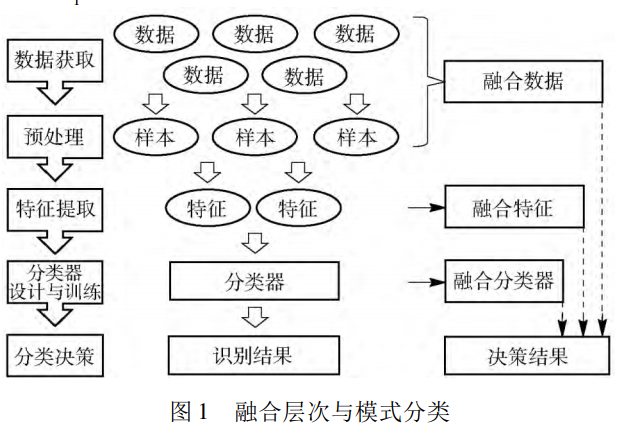## 现状 ( < 2017)

• 贝叶斯决策理论1：总结了乘法规则、加法规则、最大规则、最小规则、中位数规则、多数票规则等经典的融合规则
• 基于联合稀疏表示的特征级融合方法2:
• 稀疏核降秩回归模型3 ( SKRRR, sparse kernel reducedrank regression)
• 图像检索中的重排序( re-ranking) 问题中4
• balaba (介绍了 视频处理的涉及特征融合的 算法 雨女无瓜)
• 总结特征融合的研究现状：
• 基于贝叶斯决策理论的算法， 它的基础是统计推断和估计理论， 这类算法目的在于求解联合概率密度;
• 基于稀疏表示理论的算法， 这类算法通常与最优化理论相结合， 将
特征融合问题转化为优化问题， 进而求解联合稀疏表示来达到融合目的;
• 基于深度学习理论的算法， 这种算法的主要思想是在深度卷积神经网
络中加入特征融合过程， 以改进网络模型．

## 算法

### 基于贝叶斯理论的特征融合算法

#### 决策级水平融合(*)

• 该理论先从决策级发展出来的，一句话，取后验概率最大的类别标签作为最终 类别
• x：分类器输出结果；w：分类标签
x → ω j ,  if  F ( ω j ) = max ⁡ k = 1 , ⋯   , C P ( ω k ∣ x ) P ( ω k ∣ x 1 , ⋯   , x M ) = p ( x 1 , ⋯   , x M ∣ ω k ) p ( ω k ) p ( x 1 , ⋯   , x M ) \begin{aligned} & x \rightarrow \omega_{j}, \\ \text { if } F\left(\omega_{j}\right) &=\max _{k=1, \cdots, C} P\left(\omega_{k} \mid x\right) \end{aligned} \\ P\left(\omega_{k} \mid \boldsymbol{x}_{1}, \cdots, \boldsymbol{x}_{M}\right)=\frac{p\left(\boldsymbol{x}_{1}, \cdots, \boldsymbol{x}_{M} \mid \omega_{k}\right) p\left(\omega_{k}\right)}{p\left(\boldsymbol{x}_{1}, \cdots, \boldsymbol{x}_{M}\right)}
• 防止除0，直接取对数：
Z → ω j  if  F ( ω j ) = max ⁡ k = 1 [ ( 1 − M ) P ( ω k ) + ∑ i = 1 M P ( ω k ∣ x i ) ] \begin{array}{c} Z \rightarrow \omega_{j} \\ \text { if } F\left(\omega_{j}\right)= \\ \max _{k=1}\left[(1-M) P\left(\omega_{k}\right)+ \sum_{i=1}^{M} P\left(\omega_{k} \mid x_{i}\right)\right] \end{array}
• 先验后验近似相等情况下，认为：
P ( ω k ∣ x k ) = P ( ω k ) ( 1 + δ k i ) P\left(\omega_{k} \mid \boldsymbol{x}_{k}\right)=P\left(\omega_{k}\right)\left(1+\delta_{k i}\right)
• 搞一堆公式，最后发现，近似取 P ( ω k ) P\left(\omega_{k}\right) 最大的标签就完事了！

#### 特征级水平融合

• 由于分类器独立性假设与先验概率和后验概率近似相等假设都有相应的成立条件， 在各种应用情景中不一定完全成立， 尤其是 分类器独立性假设在许多分类问题中都难以成立．
• 线性特征依赖模型5：该算法将特征量的每个维度都看作一个分类器， 致使运算时间复杂度较高， 有待改进． 评价：卵用没有

### 基于稀疏表示理论的特征融合算法

• 稀疏表示理论是信号处理领域的一种基础理论， 它的基本思想是使用数据稀疏性这一先验知识，从一个超完备字典中找到尽可能少的原子对目标信号进行线性表示．
min ⁡ ∥ x ∥ 0  s.t.  A x = b \begin{array}{l} \min \|\boldsymbol{x}\|_{0} \\ \text { s.t. } \boldsymbol{A x}=\boldsymbol{b} \end{array}
• A是字典，b是特征
• 学习 稀疏表示矩阵x
• 显然稀疏表达的效果好坏和我们用的字典有着密切的关系。字典分两类，一种是预先给定的分析字典，比如小波基、DCT等，另一种则是针对特定数据集学习出特定的字典。这种学出来的字典能大大提升在特定数据集的效果。

# 偏传统的特征融合方法6

• 下图这种方法，可以作为毕设中 偏机器学习特征融合算法的 补充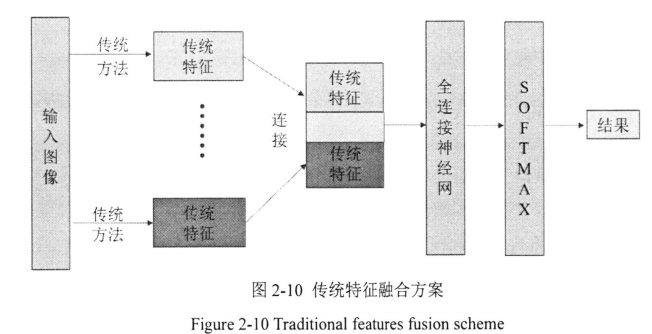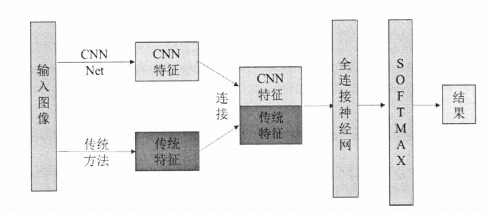• 直接用全连接层，这种方法需要你手动框选出一些目标区域和(背景/Distractor)作为样本，上述是一种 图像分类网络，只不过特征是手动设计的
• 类似的，可以采用随机森林替代全连接层

# 一些多模态特征融合的思路方法7

## 基于线性规划模型

• 线性分类器， y ( x ) = w T ⋅ x + c y(\mathbf{x})=w^{T} \cdot \mathbf{x}+c
• 建模成为一个具有稀疏性的线性规划模型，并从最大间隔原理、稀疏性和松弛变量三个方面，分别详细地阐述和分析该学习方法的原理和作用。
• 作者就是在把 SVM写了一遍 … …
• 这里还是做了一个分类器，直接就是线性分类器，权重w就是在表示 改特征的重要性！

## 基于稀疏高维映射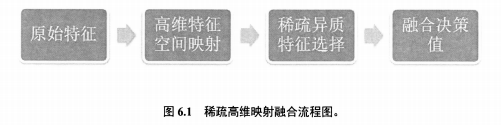• 乱七八糟，不知所云

# 字典学习 系数表示 特征融合

• 多特征联合稀疏表示人脸表情识别方法8 Page 34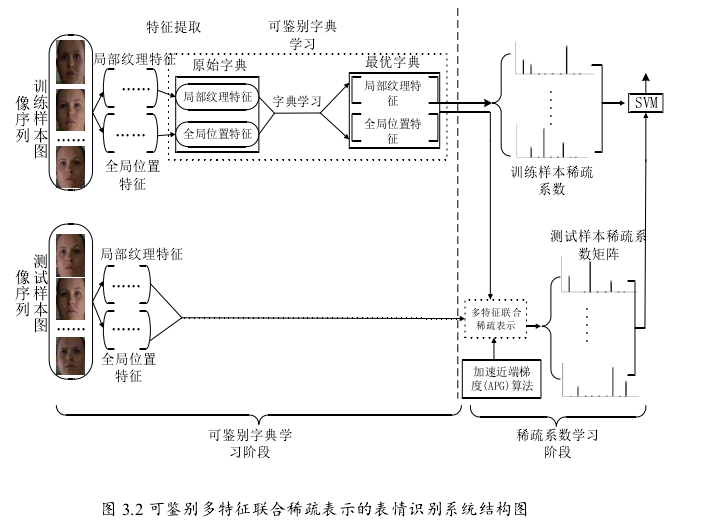1. Kittler J, Hatef M, Duin R P W, et al. On combining classifiers[J]. IEEE transactions on pattern analysis and machine intelligence, 1998, 20(3): 226-239. ↩︎

2. Shekhar S, Patel V M, Nasrabadi N M, et al. Joint sparse representation for robust multimodal biometrics recognition[J]. IEEE Transactions on pattern analysis and machine intelligence, 2013, 36(1): 113-126. ↩︎

3. Yan J, Zheng W, Xu Q, et al. Sparse kernel reduced-rank regression for bimodal emotion recognition from facial expression and speech[J]. IEEE Transactions on Multimedia, 2016, 18(7): 1319-1329. ↩︎

4. Bai S, Bai X. Sparse contextual activation for efficient visual re-ranking[J]. IEEE Transactions on Image Processing, 2016, 25(3): 1056-1069. ↩︎

5. A. J. Ma, P. C. Yuen and J. Lai, “Linear Dependency Modeling for Classifier Fusion and Feature Combination,” in IEEE Transactions on Pattern Analysis and Machine Intelligence, vol. 35, no. 5, pp. 1135-1148, May 2013, doi: 10.1109/TPAMI.2012.198. ↩︎

6. 面向目标识别的多特征融合研究与实现 ↩︎

7. 基于特征层与分数层的多模态生物特征融合 ↩︎

8. 多特征融合人脸表情识别方法研究_丁泽超 ↩︎

展开全文• 每一行叫记录、分类，每一列叫特征、字段， 二维关系表 X1~X8的值构成一个矩阵，Y构成一个矩阵，就完成输入的数据集 Y表示未来一年，糖尿病病情是否会加重 模型的改变 图片 八个特征每一个取值都和一个权重...

目录

1 模型的改变

1.1 采用Mini-Batch(N samples)的形式

2 代码的改变

2.1 构造一个多层的神经网络

2.2 代码的改变

2.2.1  数据集

2.2.2  定义多层次的神经网络函数

2.2.3  损失函数和优化器

2.2.4 训练函数

2.2.5 完整代码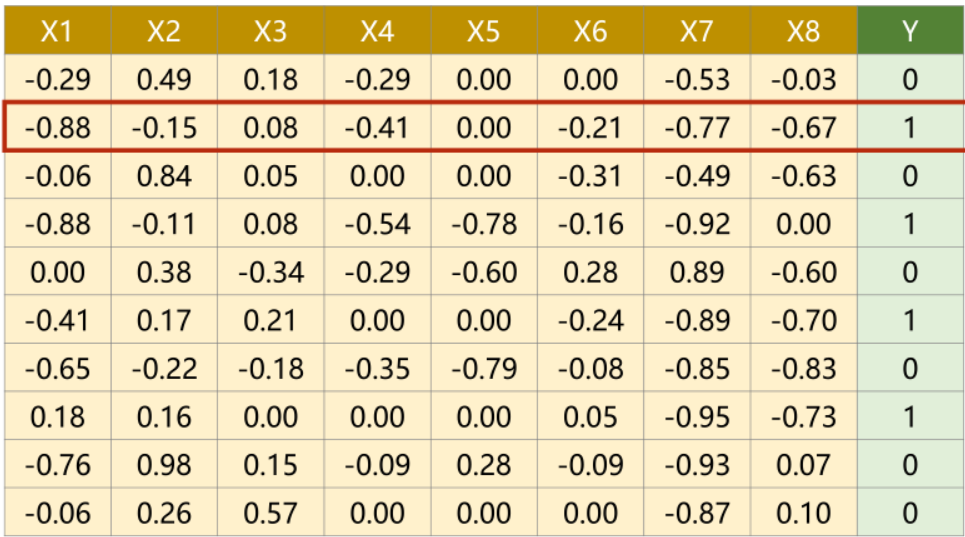本节课以糖尿病病人的数据集为例展开，如图所示是糖尿病病人的数据集，其中X1~X8表示病人8项特征的详细数据，Y表示未来一年内糖尿病病人的病情是否会加重。而我们需要做的事情就是：根据数据集中的数据，利用深度学习，让机器能够自己判断Y的取值（1表示未来一年糖尿病会加重，0表示未来一年糖尿病不会加重）。

X1~X8的值构成一个八维矩阵，Y构成一个一维矩阵，就完成输入的数据集。

注：在数据库中的表，每一行表示一个记录，每一列表示一个字段；而在深度学习的数据集中，每一行表示一个分类，每一列表示一个特征。

# 1 模型的改变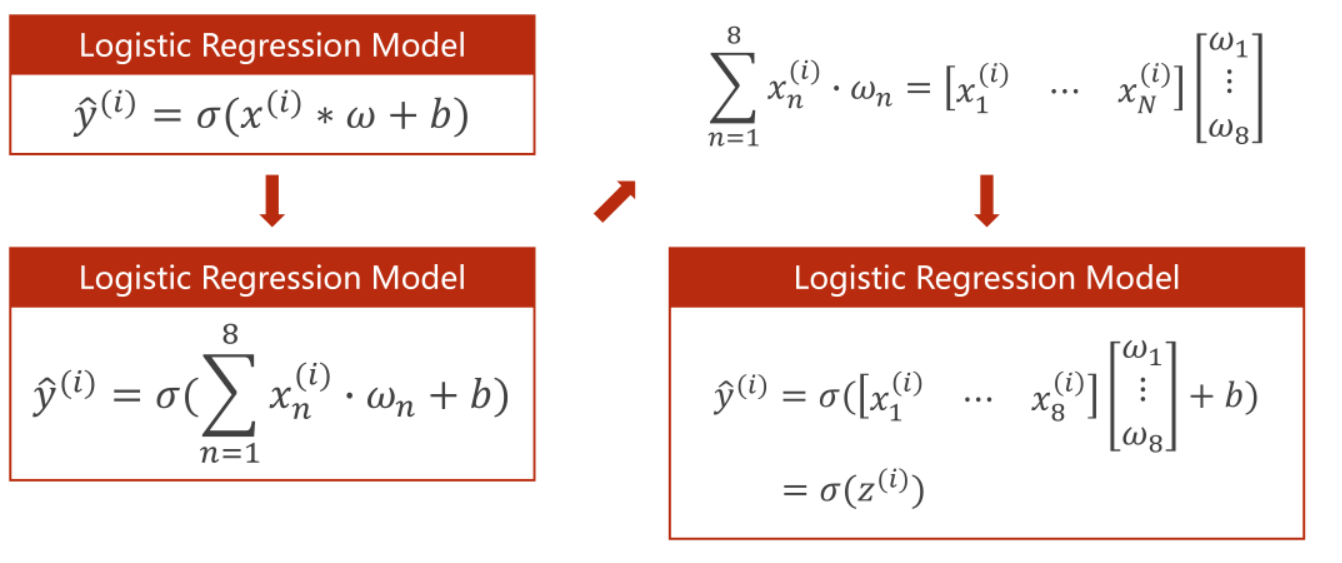在之前的学习中，因为一个样本里只有一个特征，所以只用这单个特征值乘以权重在加上偏置量，输入Sigmoid函数中，即可得到一个0到1之间的数值；

但在本节课，一个样本里有八个特征，但计算的最终结果需要是一个实数，所以将样本中的每一个特征值都都和一个权重相乘再求和，再加一个偏置量 ，最后整体再录入到sigmoid函数中，获得  值。

## 1.1 采用Mini-Batch(N samples)的形式

采用Mini-Batch的形式可以将方程运算转换矩阵的运算。

为什么要把方程运算转换成矩阵运算？

把方程运算转换成矩阵这种向量化的运算之后，可以利用计算机GPU/CPU的并行运算的能力来提高整个运算的速度。

模型的改变：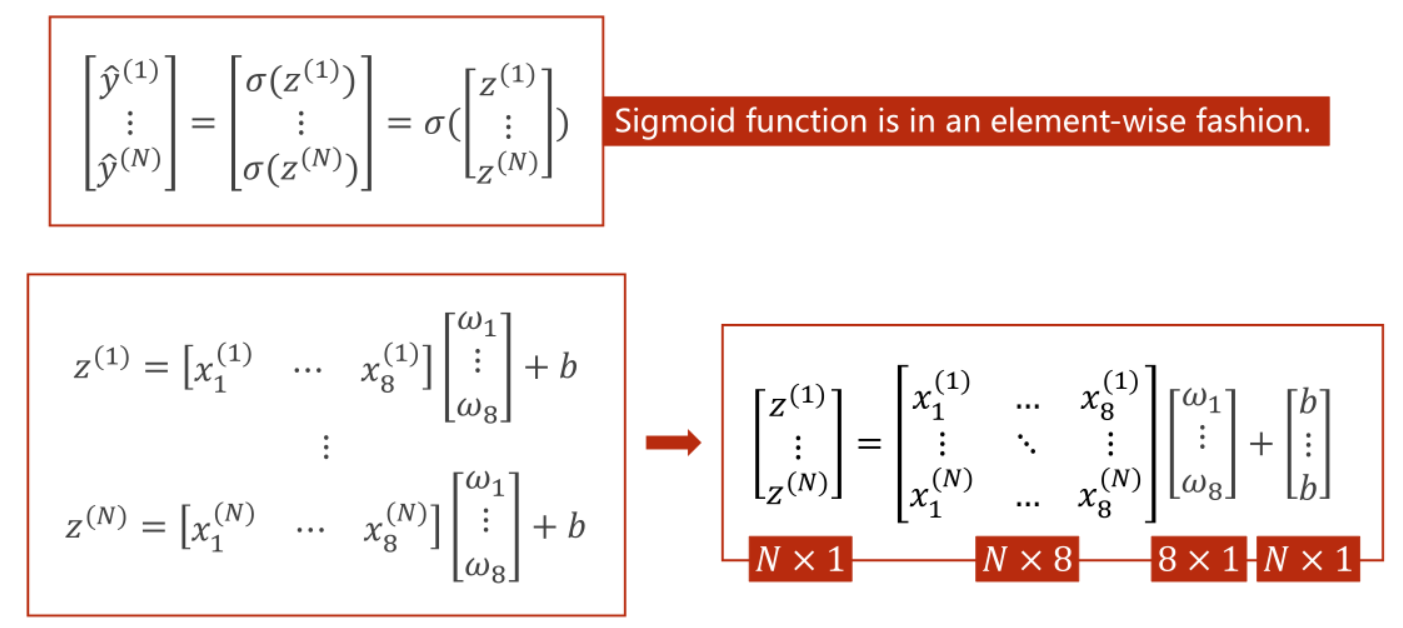Pytorch提供的Sigmoid函数是一个按照向量内每个元素计算的函数（Sigmoid function is in an element-wise dashion.）

# 2 代码的改变

## 2.1 构造一个多层的神经网络

神经网络层次叠加的过程，就是维度不断下降的过程。如下图所示，就是多层神经网络不断嵌套的过程：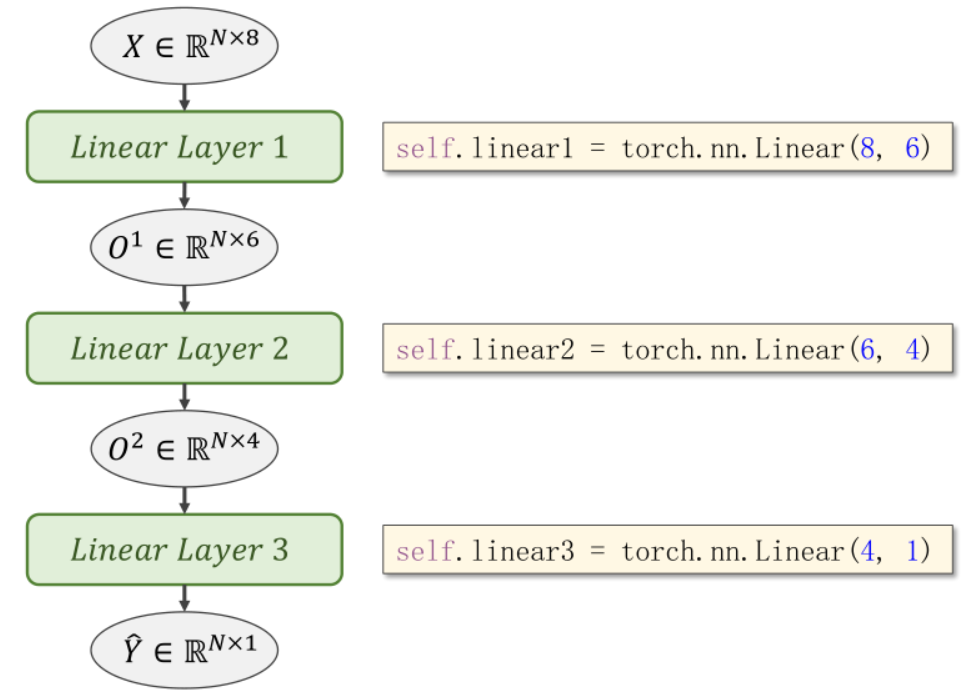神经网络的本质：就是寻找一种最优的非线性的空间变换函数。而这种非线性的空间变换函数是通过多个线性变换层，通过找到最优的权重，组合起来的模拟的一种非线性的变化。

为什么选择Sigmoid函数，因为激活函数（Sigmoid函数）可以将线性变换增加一些非线性的因子，这样我们就可以拟合一些非线性的变换。（如果每一层的神经网络只是不断的叠加线性函数的话，最终的函数还会只是一个线性函数。）

(神经网络并不是学习能力越强越好，学习能力太强，会学习数据集中的一些噪声，并不利于优化模型）

## 2.2 代码的改变

本例中并没有采用Mini-Batch的方法，依然采用的是full-batch。

### 2.2.1  数据集

将糖尿病数据集为解压文件放在与代码同目录下，如下图：

数据集下载地址：链接：百度网盘 请输入提取码   提取码：vlfd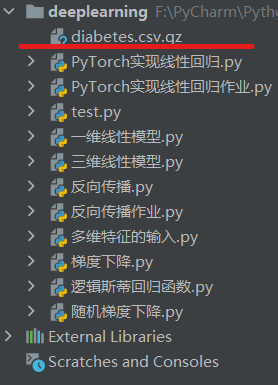具体代码如下：

xy = np.loadtxt('diabetes.csv.gz', delimiter=',', dtype=np.float32)
# 取前8列
x_data = torch.from_numpy(xy[:, :-1])
# 取最后1列
y_data = torch.from_numpy(xy[:, [-1]])

### 2.2.2  定义多层次的神经网络函数

代码如下：

class Model(torch.nn.Module):
def __init__(self):
super(Model, self).__init__()
self.linear1 = torch.nn.Linear(8, 6)
self.linear2 = torch.nn.Linear(6, 4)
self.linear3 = torch.nn.Linear(4, 2)
self.linear4 = torch.nn.Linear(2, 1)
self.sigmoid = torch.nn.Sigmoid()

def forward(self, x):
# 注意所有输入参数都使用x
x = self.sigmoid(self.linear1(x))
x = self.sigmoid(self.linear2(x))
x = self.sigmoid(self.linear3(x))
x = self.sigmoid(self.linear4(x))
return x

model = Model()

### 2.2.3  损失函数和优化器

损失函数如下：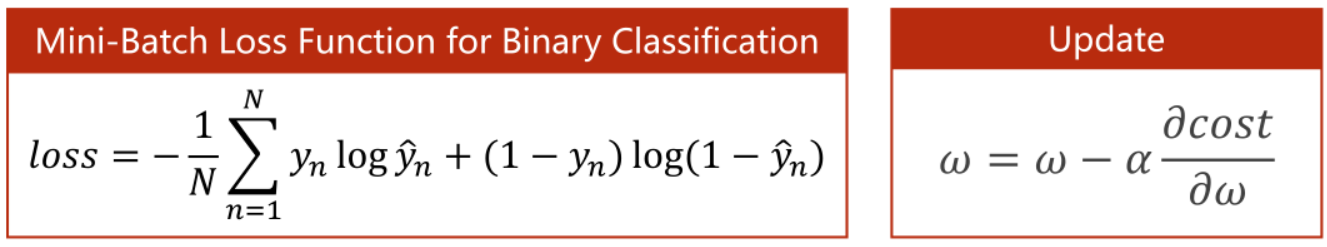损失函数依然采用交叉熵公式，但是需要取均值，所有reduction=‘mean’，课堂中老师讲的size_average=True 已经弃用。具体代码如下：

criterion = torch.nn.BCELoss(reduction='mean')
optimizer = torch.optim.SGD(model.parameters(), lr=0.1)

### 2.2.4 训练函数

具体代码如下：

for epoch in range(1000000):
y_pred = model(x_data)
loss = criterion(y_pred, y_data)
print(epoch, loss.item())

# 反馈
loss.backward()

optimizer.step()

### 2.2.5 完整代码

import numpy as np
import torch
import matplotlib.pyplot as plt

# 取前8列
x_data = torch.from_numpy(xy[:, :-1])
# 取最后1列
y_data = torch.from_numpy(xy[:, [-1]])

class Model(torch.nn.Module):
def __init__(self):
super(Model, self).__init__()
self.linear1 = torch.nn.Linear(8, 6)
self.linear2 = torch.nn.Linear(6, 4)
self.linear3 = torch.nn.Linear(4, 2)
self.linear4 = torch.nn.Linear(2, 1)
self.sigmoid = torch.nn.Sigmoid()

def forward(self, x):
# 注意所有输入参数都使用x
x = self.sigmoid(self.linear1(x))
x = self.sigmoid(self.linear2(x))
x = self.sigmoid(self.linear3(x))
x = self.sigmoid(self.linear4(x))
return x

model = Model()

criterion = torch.nn.BCELoss(reduction='mean')
optimizer = torch.optim.SGD(model.parameters(), lr=0.1)

for epoch in range(1000000):
y_pred = model(x_data)
loss = criterion(y_pred, y_data)
print(epoch, loss.item())

# 反馈
loss.backward()

optimizer.step()


部分运行截图如下：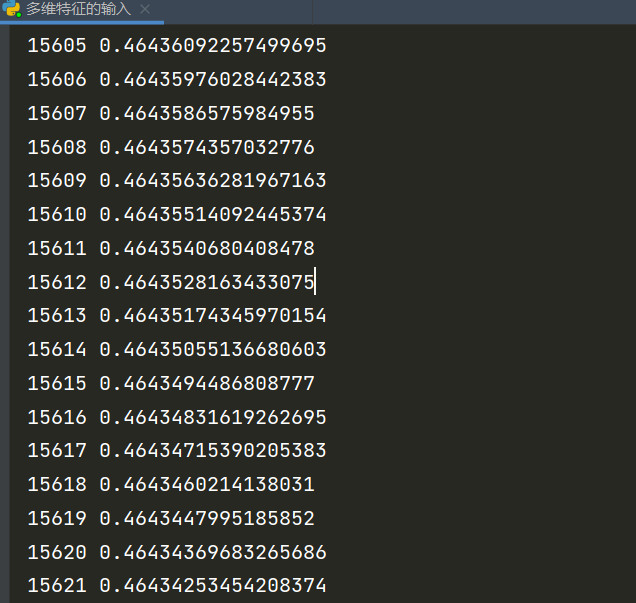展开全文深度学习 pytorch 人工智能
• 深度学习-处理多维度特征的输入 -Multiple Dimension Input-自用笔记6 多维度特征的数据集 每一行代表一个样本，每一列代表一重要特征Feature 一个样本特征多个的计算图如图所示 多个样本多个特征的计算图如图所...

# 深度学习-处理多维度特征的输入 -Multiple Dimension Input-自用笔记6

## 多维度特征的数据集

每一行代表一个样本，每一列代表一重要特征Feature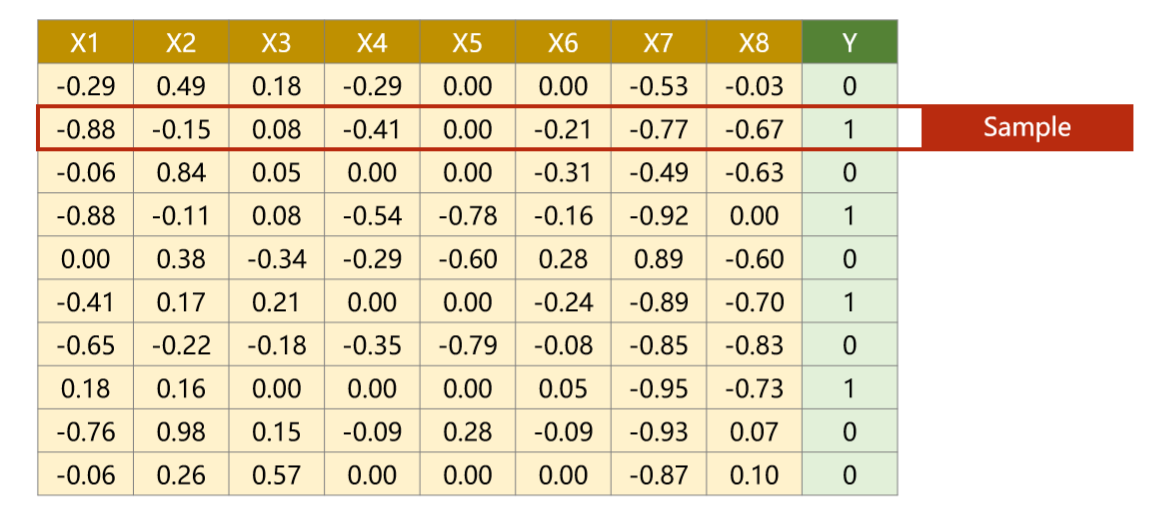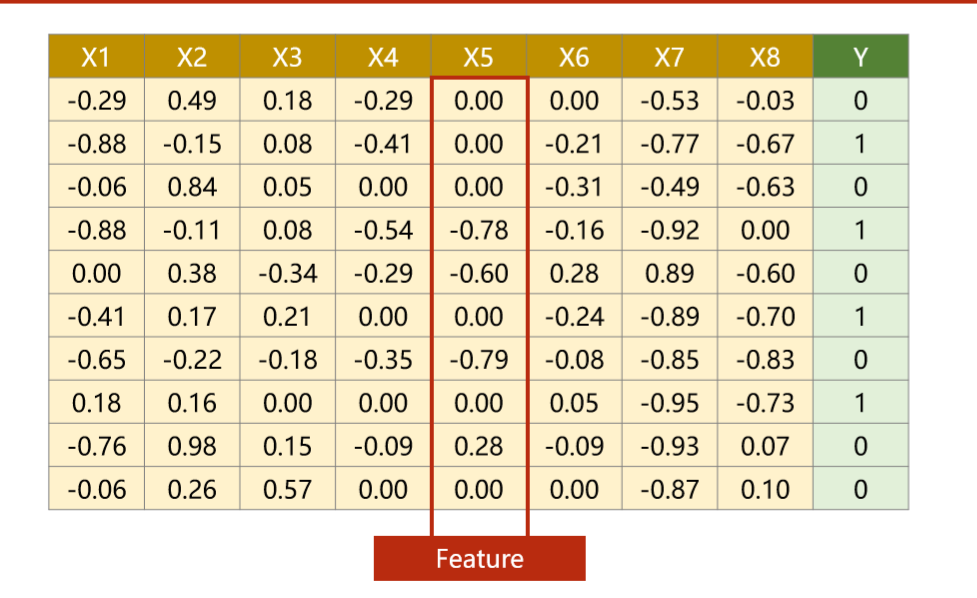## 一个样本特征多个的计算图如图所示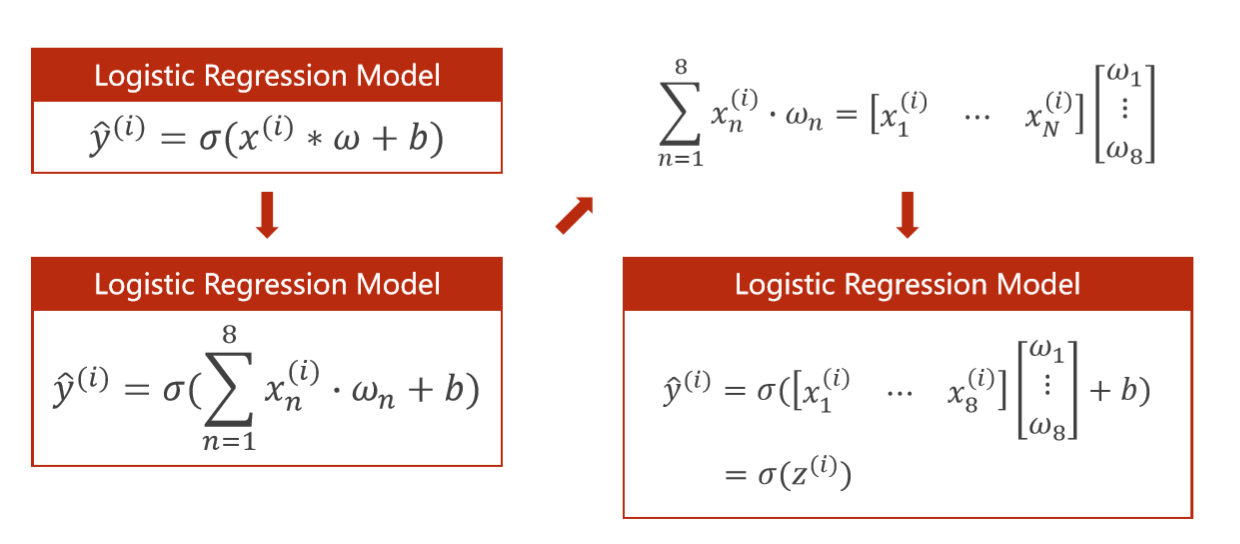## 多个样本多个特征的计算图如图所示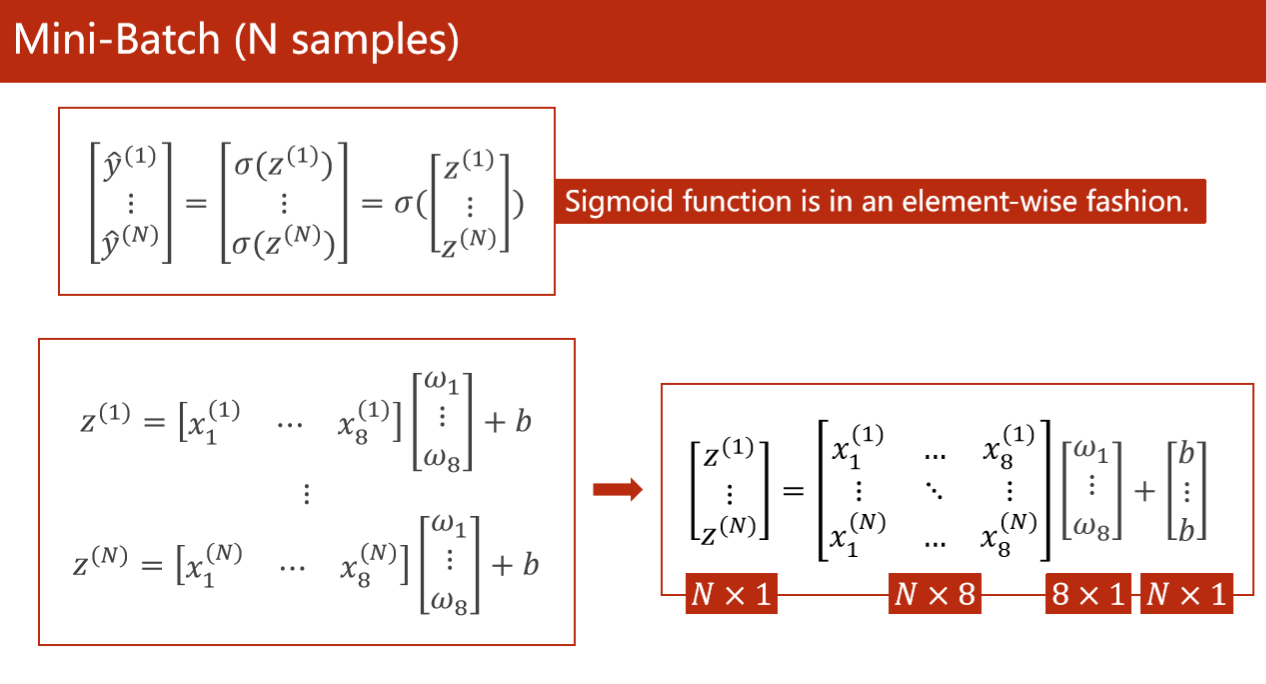模型采用一层线性函数self.linear = torch.nn.Linear(8, 1)，函数的输入的特征维度为8，输出的维度为1，如下图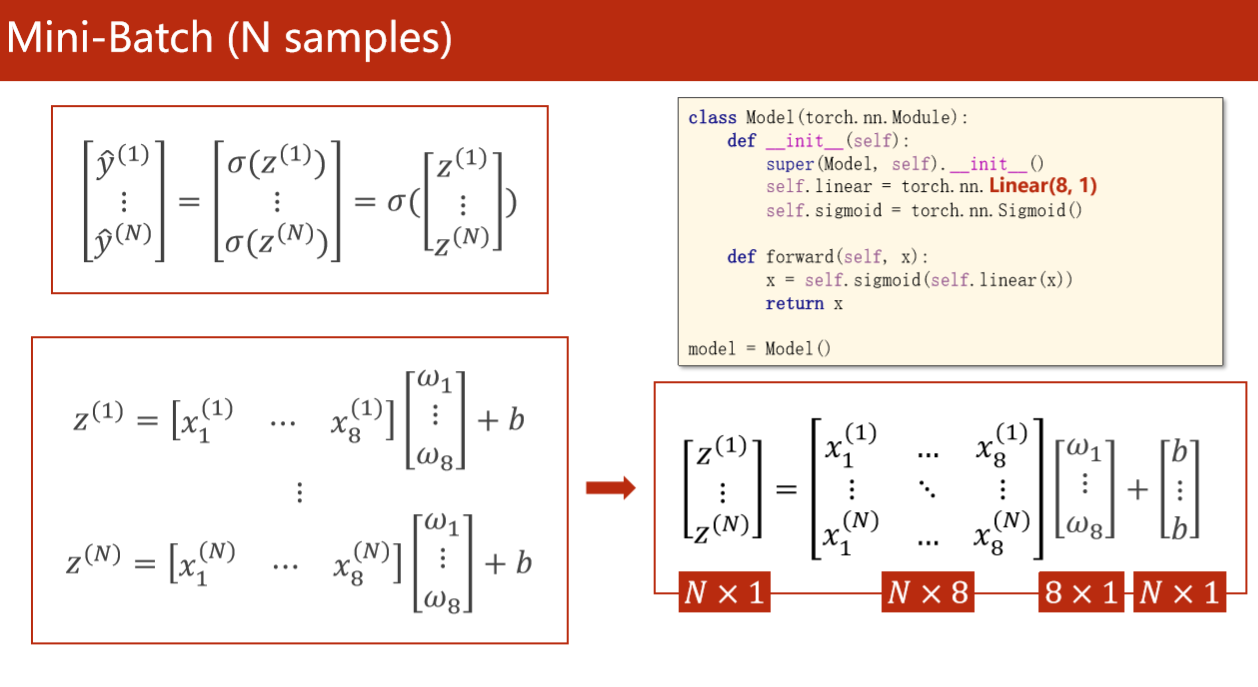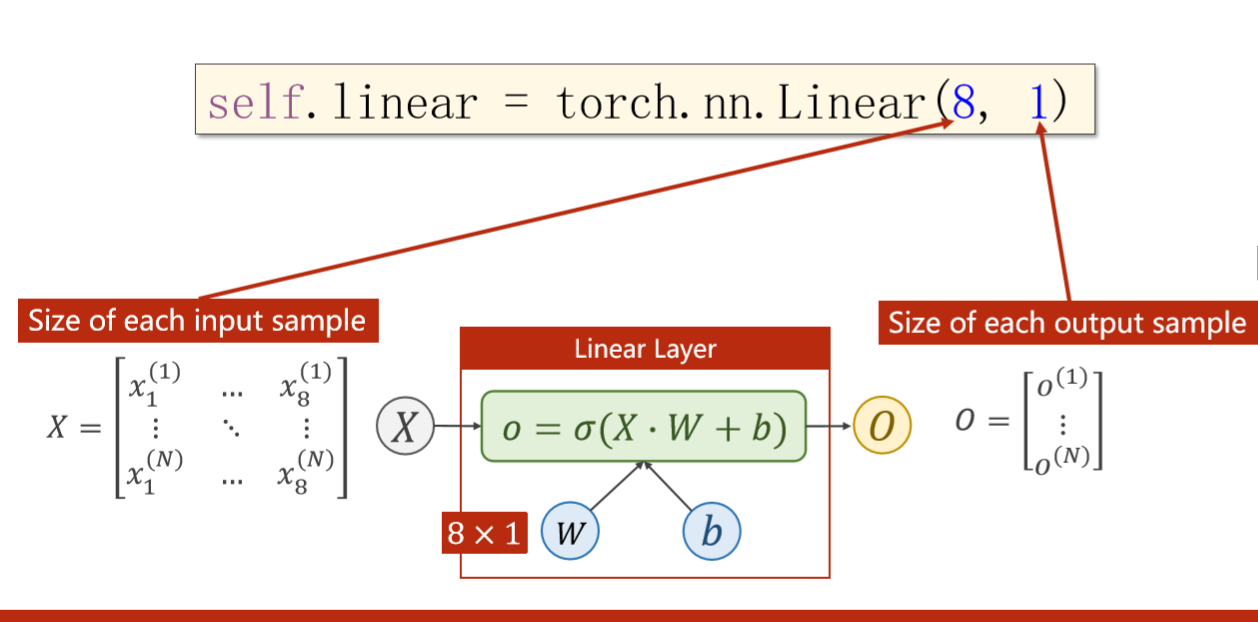若线性层函数采用输入的特征维度为8，输出的维度为2，如下图：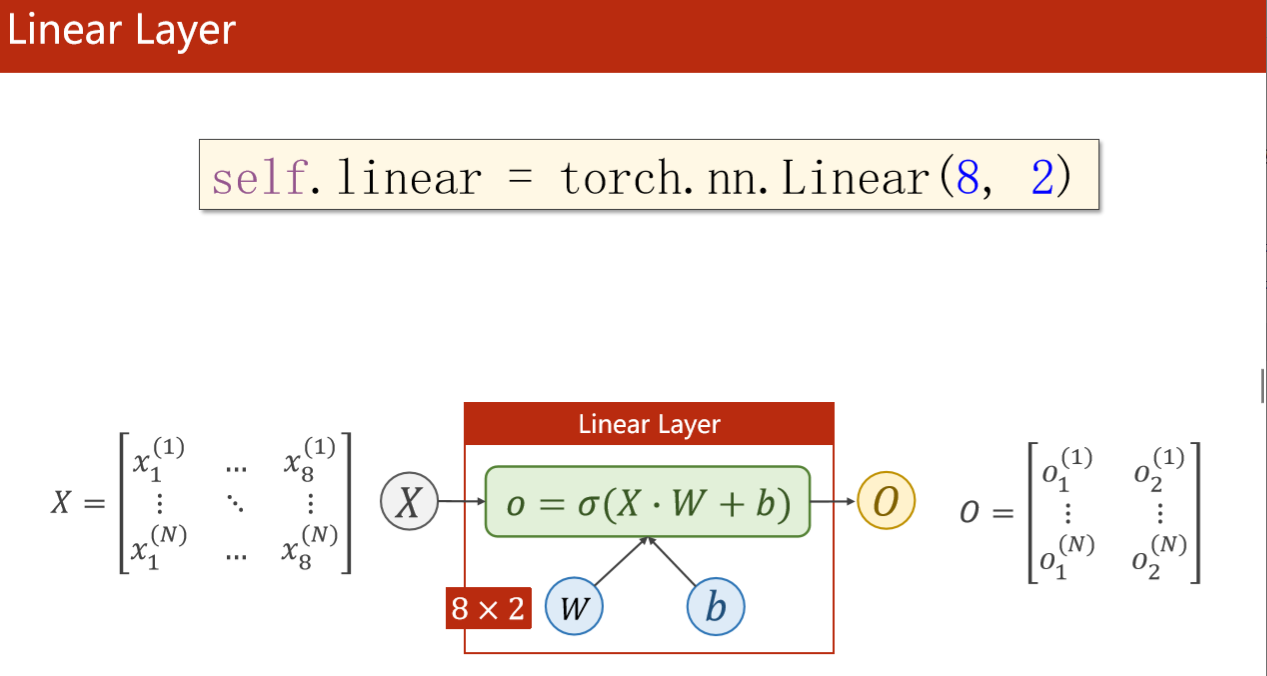若线性层函数采用输入的特征维度为8，输出的维度为6，如下图：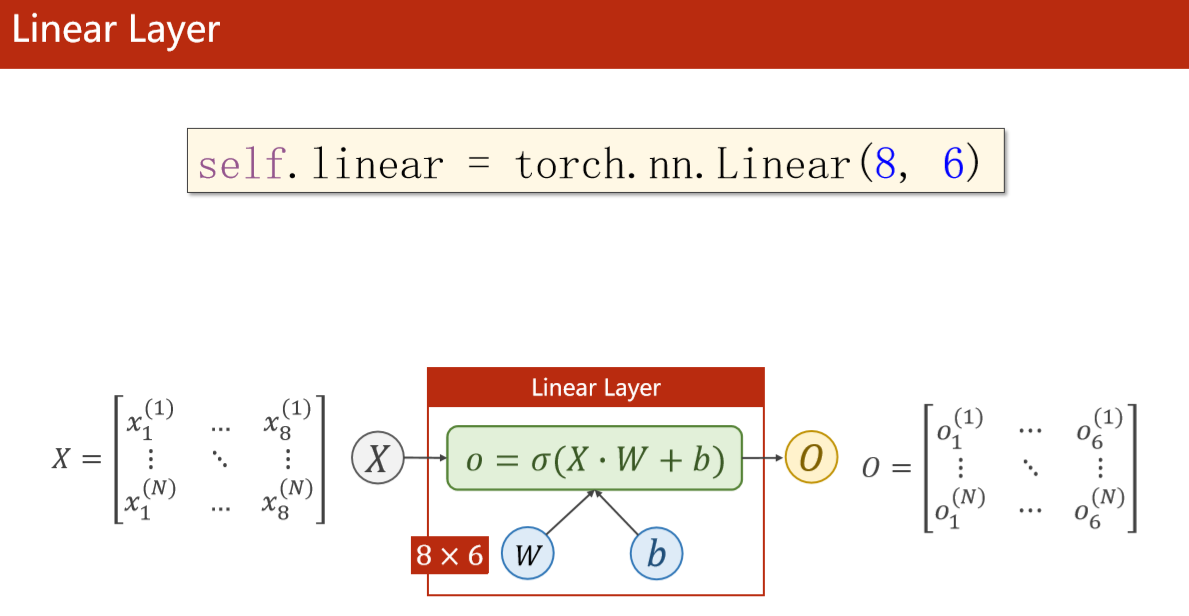## 多层神经网络的计算图

将三个一层的神经网络叠加在一起变成三层的神经网络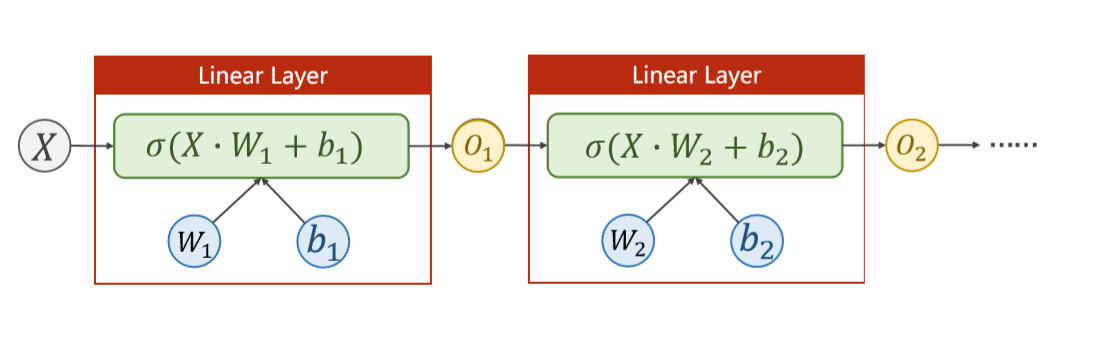例如: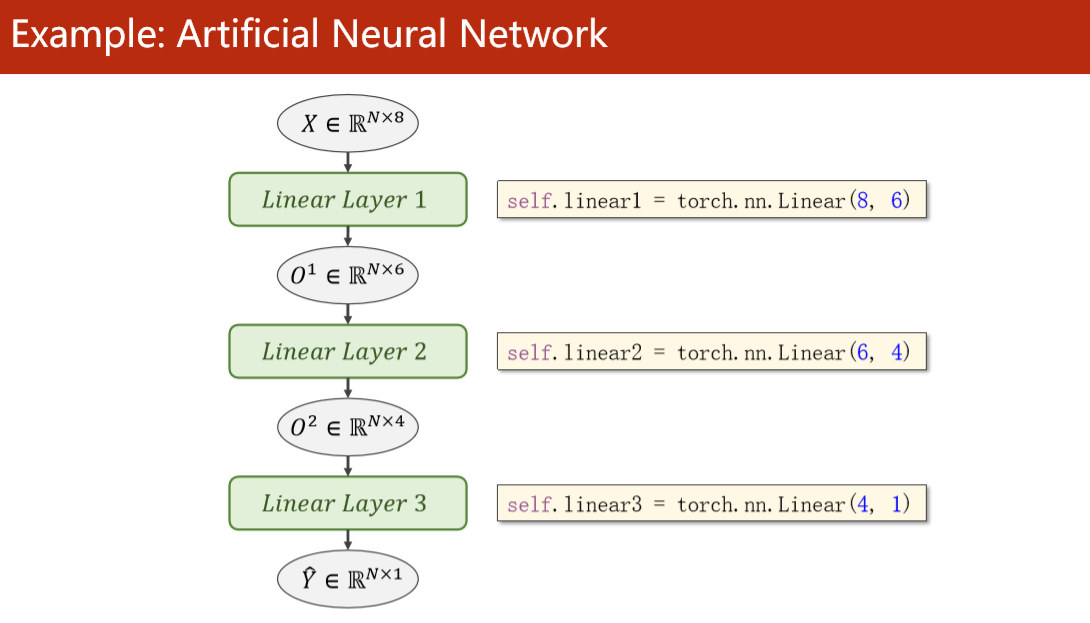## 举例子+程序代码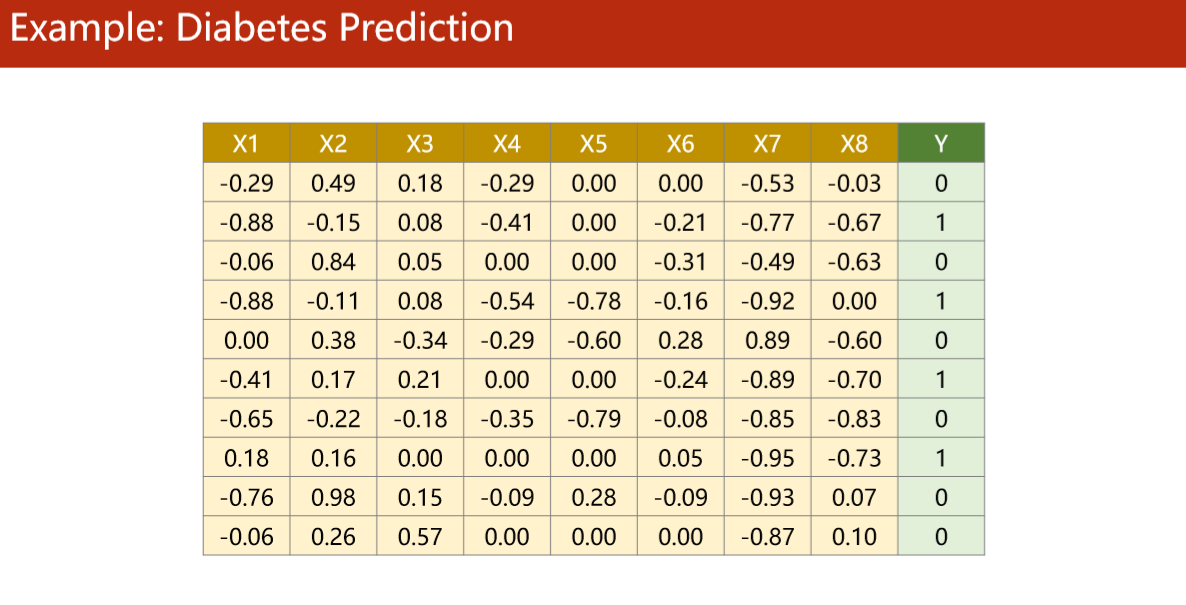1. Prepare Dataset
import numpy as np
x_data = torch.from_numpy(xy[:,:-1])
y_data = torch.from_numpy(xy[:, [-1]])

1. Define Model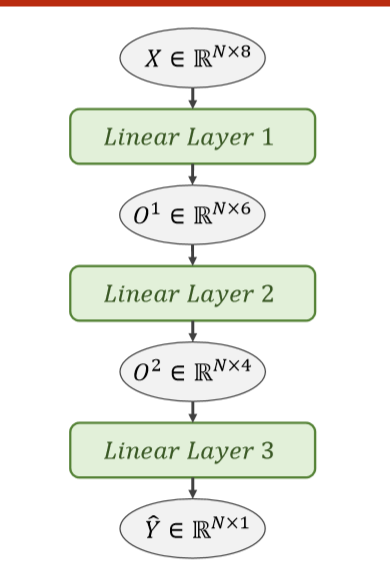代码
import torch
class Model(torch.nn.Module):
def __init__(self):
super(Model, self).__init__()
self.linear1 = torch.nn.Linear(8, 6)
self.linear2 = torch.nn.Linear(6, 4)
self.linear3 = torch.nn.Linear(4, 1)
self.sigmoid = torch.nn.Sigmoid()
def forward(self, x):
x = self.sigmoid(self.linear1(x))
x = self.sigmoid(self.linear2(x))
x = self.sigmoid(self.linear3(x))
return x
model = Model()

1. Construct Loss and Optimizer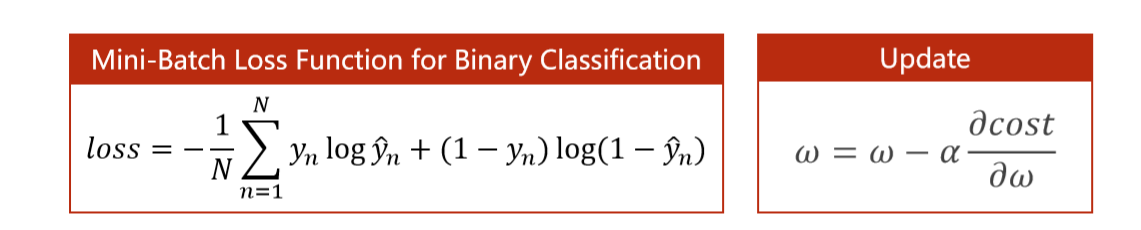代码
criterion = torch.nn.BCELoss(size_average=True)
optimizer = torch.optim.SGD(model.parameters(), lr=0.1)

1. Training Cycle
代码
for epoch in range(100):
# Forward
y_pred = model(x_data) l
oss = criterion(y_pred, y_data)
print(epoch, loss.item())
# Backward
loss.backward()
# Update
optimizer.step()


## 注意：（激活函数种类很多，采用RuLU激活函数时需要注意）

现在非常流行使用RuLU激活函数，但是RuLU存在的问题是当激活函数的输入为小于0时，激活函数的梯度就变为0，不会继续更新维度，所以采用RuLU需要注意。
一般如果做分类，采用RuLU激活函数都是在前面的层数，最后一层激活函数不要使用RuLU，一般会采用sigmoid的。

import torch
import numpy as np
import torch.nn.functional as F
x_data = torch.from_numpy(xy[:,:-1]) #取全部行和除了最后一列的全部列的数据
y_data = torch.from_numpy(xy[:, [-1]])#只取最后一列的数据，并列为一列矩阵，torch.from_numpy该语句的意思是将数组转换成tensor类型。
class Model(torch.nn.Module):
def __init__(self):
super(Model, self).__init__()
self.linear1 = torch.nn.Linear(8, 6)
self.linear2 = torch.nn.Linear(6, 4)
self.linear3 = torch.nn.Linear(4, 1)
self.activate = torch.nn.ReLU()
def forward(self, x):
x = self.activate(self.linear1(x))
x = self.activate(self.linear2(x))
x = F.sigmoid(self.linear3(x))
return x
model = Model()
# ------------------------------------------------------- #
criterion = torch.nn.BCELoss(size_average=True)
optimizer = torch.optim.SGD(model.parameters(), lr=0.1)
# ------------------------------------------------------- #
for epoch in range(10000):
# Forward
y_pred = model(x_data)
loss = criterion(y_pred, y_data)
print(epoch, loss.item())
# Backward
loss.backward()
# Update
optimizer.step()

展开全文深度学习 pytorch
• 因此，降低特征矩阵维度，也是必不可少的，主成分分析就是最常用的降维方法，在减少数据集的维度的同时，保持对方差贡献最大的特征，在sklearn中，我们使用PCA类进行主成分分析。 主成分分析（Principal Components ...
• 如果要素和要素2包含同一批图像的特征,那么要素[i]与每个i的要素2 [i]的图像相同,那么使用numpy函数将要素分组到单个数组中是有意义的拼接()：newArray = np.concatenate((features, features2), axis=3)其中3是阵列...
• K-means聚类算法c语言实现。样本数据从文件读入，支持任意维数数据和任意k值（k当然要小于样本数）.原创原创！
• 随着气候雾霾污染问题...通过实验模拟绝缘子在雾霾条件下的工作状态,测量绝缘子雾霾天的泄漏电流,提出利用小波分析和关联维度特征值对雾霾天的泄漏电流进行对比分析。同时,给出关联维度分析方法的缺陷以及改进办法。
• 对比2个工作日上午时段的交通拥堵时间百分比、持续时间特征指标，引入残存函数描述路段拥堵持续时间分布概率，采用曲线拟合后得到Log-Logistic模型可以更好地描述拥堵持续时间残存函数曲线，并对比不同路段的拥堵...
• 一句话：维度是一个数字，表征每个样本中特征的数量。 机器学习中，对于数据一般用n∗mn*mn∗m矩阵表达，nnn表示样本（sample）数量，mmm表示特征（attribute/feature/variable）数量。如下图，即是4∗24*24∗2的...
• 输入全连接层，一定要把tensor调整为二维的，比如[None, ...输入LSTM之前，要把维度调整为三维的，比如[None, 20,60]，None是batch_size随意的意思，20代表循环输入20个时间戳，60代表每个时间戳有60个特征属性。 ...tensorflow
• 问题：如何经过convTransposed1d输出指定大小的特征？ import torch from torch import nn import torch.nn.functional as F conv1 = nn.Conv1d(1, 2, 3, padding=1) conv2 = nn.Conv1d(in_channels=2, out_...
• 对于机器学习任务，我正在寻找一种方法来合并两个不同维度特征矩阵，这样我就可以把它们都提供给估计器。我不能使用scipy合并方法，因为这些方法需要兼容的形状。我可以使用numpy合并方法，但当我实际尝试分割数组...
• 目前似乎没有不同维度的数据的相似度，只能通过一些方法来做，下面是我的尝试，仅供参考。 考虑到人脸识别及验证中其实不同图像人脸也不一样大，是如何做的识别？？ 这个是有图像的resize存在，然后经过模型得到...相似度
• 多尺度特征融合：像SSD（Single Shot Detector）采用这种多尺度特征融合的方式，没有上采样过程，即从网络不同层抽取不同尺度的特征做预测，这种方式不会增加额外的计算量。 特征金字塔（FPN）：顶层特征通过上采样...计算机视觉
• 实际问题中，样本的属性就是样本的特征向量，所以样本的特征向量维度越高，张成的样本空间就越大，如果样本的特征向量是二维的（x, y）,则样本空间是是一个二维空间，即一个平面，如果特征向量是三维的（x, y, z）,...机器学习
• 针对当前输电线路故障诊断的需求，结合智能电网运行中产生的大量结构多样、来源复杂的数据，将这些大数据归类于不同维度，设计了基于多维度数据融合的输电线路故障智能诊断系统。对多维度的诊断结果融合架构、融合...
• 一、介绍本篇文章，我们将讨论所谓的“维度灾难”，并解释在设计一个分类器时它为何如此重要。...要做到这一点，我们首先需要考虑猫、狗的量化特征，这样分类器算法才能利用这些特征对图片进行分类。例如我们可以通机器学习
• 做机器学习实验K-means时，我想把每个聚类（cluster）当成每个元素放入np.array中，而每个聚类是一个矩阵，矩阵的行数是该类样本点的个数，矩阵的列数是每一个样本点的特征数（即维度）。 ***我希望得到的***...python numpy
• % 计算协方差需要计算均值,协方差矩阵是计算不同维度间的协方差，要时刻牢记这一点。样本矩阵的每行是一个样本，每列为一个维度，所以我们要按列计算均值。 %计算dim1与dim2，dim1与dim3，dim2与dim3的协方差： ...
• ## 事实表与维度表

千次阅读 2021-08-26 15:49:19
文章目录前言一、事实表1.2、事实表的特征1.3、事实表的类型1.3.1、交易事实表1.3.2、快照事实表1.3.3、积累事实表1.4、它如何在数据仓库中工作？1.5、事实表的优点二、维度表2.1、维度表类型2.1.1、SCD（缓慢变化维...数据仓库
• 对于Encoder来说，q, k, v初始化下是由x乘以一个W得到的，比如说现在是8头自注意力机制，经过Embedding之后升高的维度d_model=64，那么Encoder第一个Sublayer得到的q,k,v的维度初始情况下是[batch_size, in_seq_len,...自然语言处理 pytorch
• ## 维度灾难

千次阅读 2018-09-05 10:26:50
在机器学习中我们需要设定模型的特征值，而每一个特征值又含有不同的数值代表不同类别。在机器学习的过程中，为了更好的区分数据，我们往往会增加模型的特征维度（即特征值得个数），如下图所示，...机器学习
• 1 维度表的定义在维度建模中，通常将指标的度量称之为“事实”，将产生度量的环境称之为“维度”。将描述同一个业务实体的的多个维度列组合在一起，就是常说的“维度表”。维度表是用户分析数据的窗口，其提供了事件...
• 在本文中，我们分析了卖方声誉的不同维度如何影响电子市场中的定价能力。 考虑到买主的信任和卖主的定价能力之间的相互作用，我们使用文本挖掘技术从信誉系统上发布的反馈中识别和构建重要性维度。 通过根据它们所...
• 在实际应用中综合利用各种不同特征进行图像检索有很多优点。其中最主要的是： 1）可以达到不同特征之间的优势互补效果。例如，在颜色特征的基础上增加爱纹理特征既能弥补颜色特征缺乏看见空间分布信息的不足，又...
• 对于刚接触神经网络的人，理解了网络层级之后就是要自己搭建网络，设置参数，这个时候就需要计算参数,特征图尺寸，输出维度等，这个其实看一遍流程，动手测试一下就懂了，所以我也在这里记录一下。 不同的框架，...神经网络 理论学习...